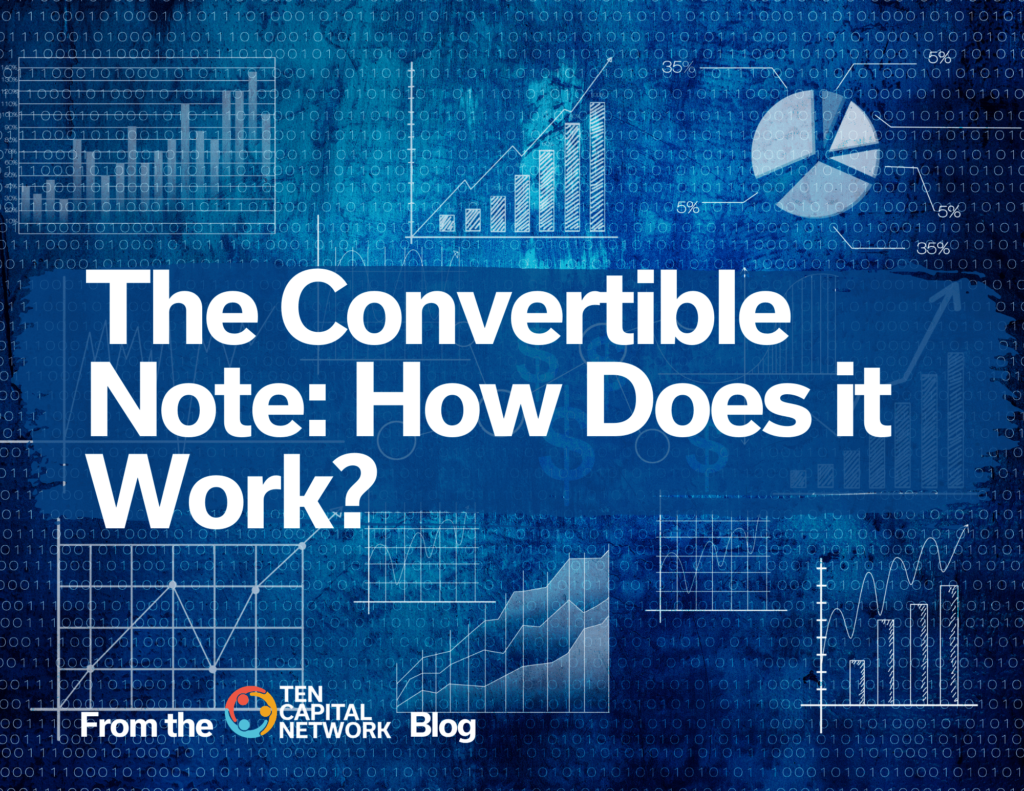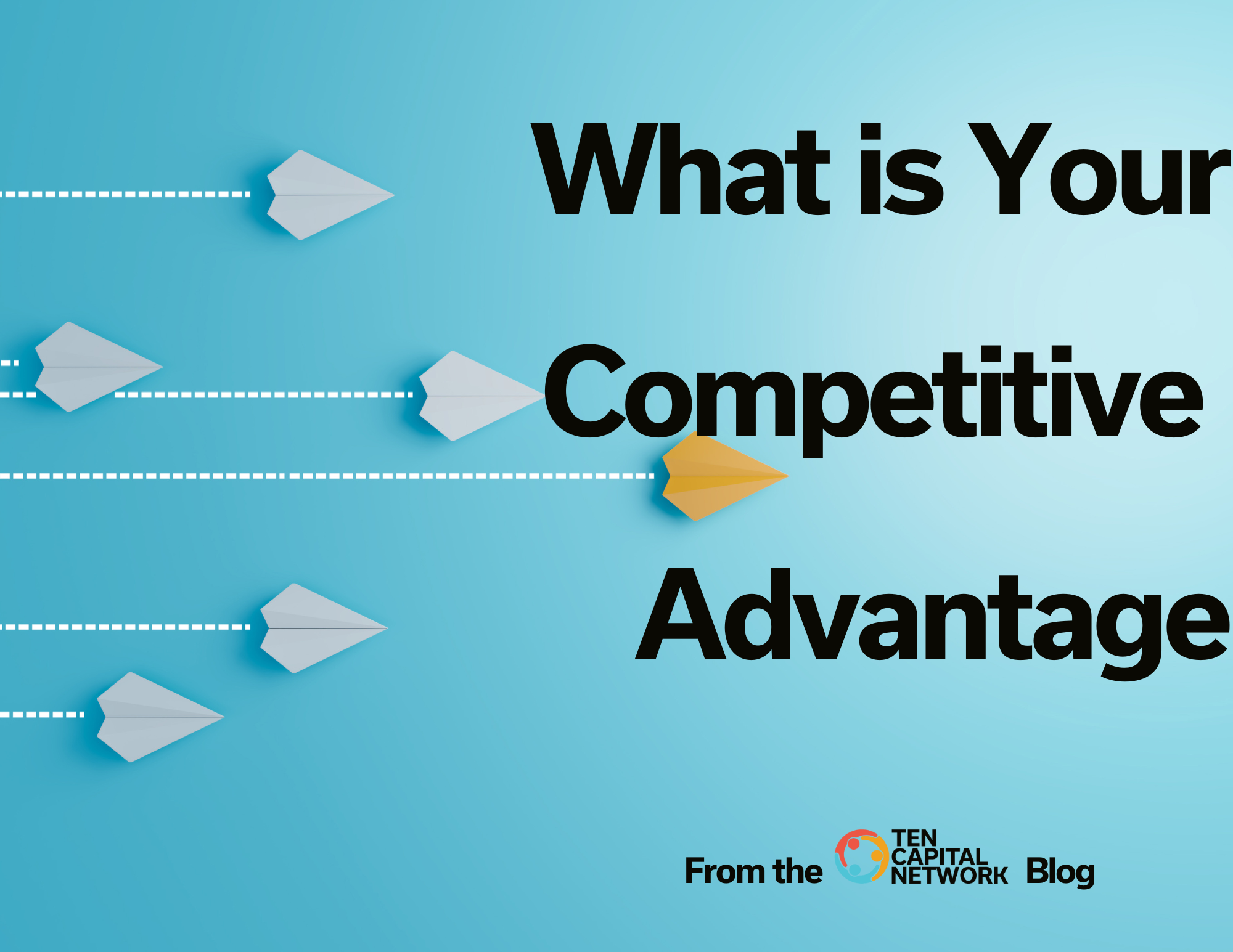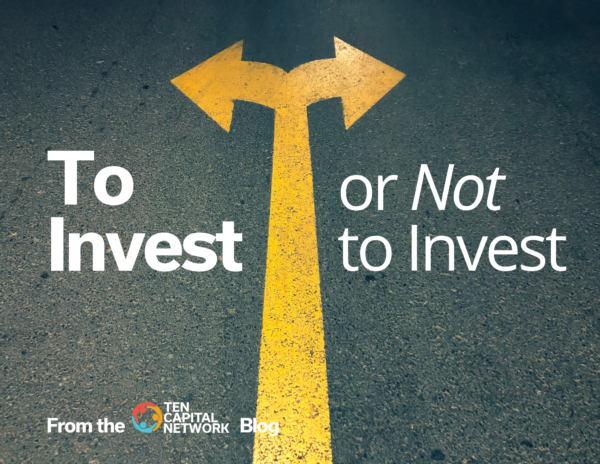Startup Funding# The Convertible Note: How Does it Work?1 min read A commonly used investment tool for funding startups is the Convertible Note.

##### What is a Convertible Note?

A short-term debt instrument that converts into equity later. If the issuer wants a debt instrument without conversion to equity, a promissory note would be a better option.

With a convertible note, the investor receives accruing interest while holding the note.

##### Why Use a Convertible Note?

It works well for seed-stage startups as it removes the burden of a complex equity-based terms sheet which requires details on control and boards, and avoids issues of dilution and taxes.

It’s easy to set up compared to most equity terms sheets which can be quite costly to develop since valuation must be negotiated and set at the time of signing.

The convertible note also works well for investors who want to invest relatively small amounts. Investors seeking to make large investments typically want valuation set, board seats determined, and control provisions set which often requires an equity terms sheet.

The convertible note is a useful tool for early-stage startups where there are still many unknowns about the deal.

##### The Three Components:

A Convertible Note has three components: the interest rate, discount rate, and cap rate.

1. The interest rate determines the annual interest that will accrue. The interest is not meant to be paid out monthly or quarterly like a bank loan but will convert to equity later along with the principle.
2. The discount rate is the amount of additional equity the investor will receive when the note converts to equity as compensation for investing early.
3. The cap rate determines how much equity the investor will receive upon conversion.
##### How Does it Work?

The conversion from debt to equity is usually based on a future financing round. If there is no follow-on financing round, then the note often sets a time limit (say 5 years) at which point it will convert at the cap rate.

The interest rate is typically a simple interest rate. If the price per share is \$4 and the interest rate is 10%, then the investor receives \$4*.10= \$0.40/share in the form of interest.

The discount rate gives a reduced price to the convertible note holder. If the price per share is \$4 and the discount is 15%, then the note holder receives their share at a price of (\$4 * (1-.15)) = \$3.40.

The cap rate sets a maximum limit at which the convertible note can convert to equity. For example, if the cap rate is \$3M and the next round of financing comes in at \$5M, and the share price is \$4.  Then the price per share to the convertible note holder is \$2.40. (3/5=.6; \$4*.6=\$2.4).

Read more about Convertible Notes and take our Note Calculator here: https://www.startupfundingespresso.com/note-calculator/Hall T. Martin is the founder and CEO of the TEN Capital Network. TEN Capital has been connecting startups with investors for over ten years. You can connect with Hall about fundraising, business growth, and emerging technologies via LinkedIn or email: hallmartin@tencapital.group

## Recent blog## Team Due Diligence

1 min read No one wants to invest in a startup that is doomed to fail; enter Team Due Diligence. “What we …## The Importance of Diversity in Your Portfolio

1 min read The Importance of Diversity in Your Portfolio According to a Harvard Business Review study on increasing diversity in venture …